# Line of Fit & Line of Best Fit: Definitions & Equations

Instructor: David Broy

Dave has taught college-level math, physics, computer, and electronics courses and has a Master's Degree in Electrical Engineering.

In this lesson, learn the definition of the line of best fit and how to calculate it for your data in a scatter plot. We'll cover slope and y-intercept formula, and go through an example to see it all in action.

## Observing Trends in Scatter Plots

One of the more challenging steps in the scientific method is the analysis. It's relatively straightforward to collect data, but then you have to answer the question, ''Now what do I do with it?''

One way to observe trends in collected data is to create a scatter plot, a two-dimensional graph of y versus x, where x and y are two of the quantities in your data set.

What you hope to see in a scatter plot is the relationship between x and y, in which the value of quantity y (the dependent variable) in some way depends on the value of quantity x (the independent variable). Let's take a look at an example plot where there is a visible, underlying relationship: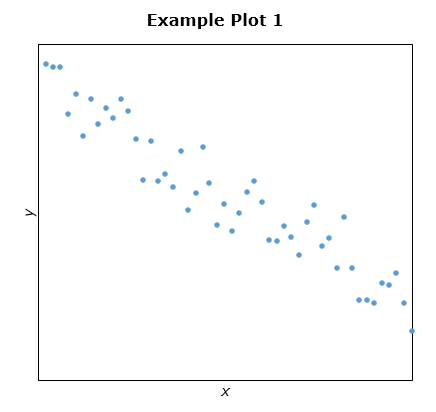In Example Plot 2, it appears that x and y are not related to each other: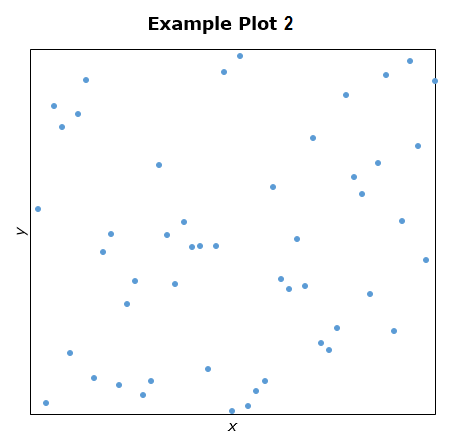## Line of Fit

When there is a relationship between two variables, quite often it's a linear relationship, and your scatter plot will be similar to Example Plot 1, where it appears the underlying trend in the data is a straight line.

You could eyeball the graph and, with a pencil and a straightedge, sketch in the line where it appears to fit the data, estimate its slope and y-intercept, and be satisfied that your estimate is close enough to meet your needs. But if you are looking for something better, it is worth the extra effort to obtain the mathematical definition of the line that most accurately represents the data, the line of best fit.

## Line of Best Fit

Also referred to as 'trend line', the line of best fit is the line for which the sum of the squares of the residual errors between the individual data values and the line is at its minimum—which is just a fancy way of saying it is the best possible straight line that fits your data.

Slope and y-intercept are the two numbers needed to define the equation of a line in slope-intercept form, y = mx + b.

So there are two formulas needed to define the line of best fit, one for the slope: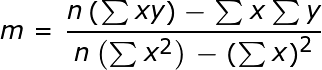and one for the y-intercept: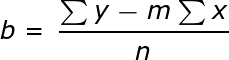where:

• m is the slope
• b is the y-intercept
• n is the number of points in the scatter plot
• x is the set of x-values of the points
• y is the set of y-values of the points

Note there are four distinct summations which must be calculated:

1. Σx (adding up the x-values)
2. Σy (adding up the y-values)
3. Σx2 (squaring each x-value and then adding up the x2 values)
4. Σxy (multiplying each x-value by its corresponding y-value and then adding up the xy values).

Also note the difference between (Σx)2 (taking the already calculated Σx and squaring it) and the previously defined Σx2.

This might seem like a lot of number crunching to you, and it really is, especially for larger data sets. So there are, of course, calculators, both handheld and online, which will do the number crunching for you. However, it is probably worth the extra effort to walk through these calculations yourself on a couple smaller data sets just to get that deeper understanding and appreciation of it all. (Just like baking a cake from scratch as opposed to using a box mix or just picking one up at your local bakery.)

## Example

Suppose you want to know the relationship between the amount of time a student spends studying for a test, and the grade he or she receives on that test. You are granted permission from a local high school to access Mr. Mann's Tenth Grade History Class in order to obtain the information you need. By the end of the day, you have the number of hours spent studying and the exam score for each of the 30 students: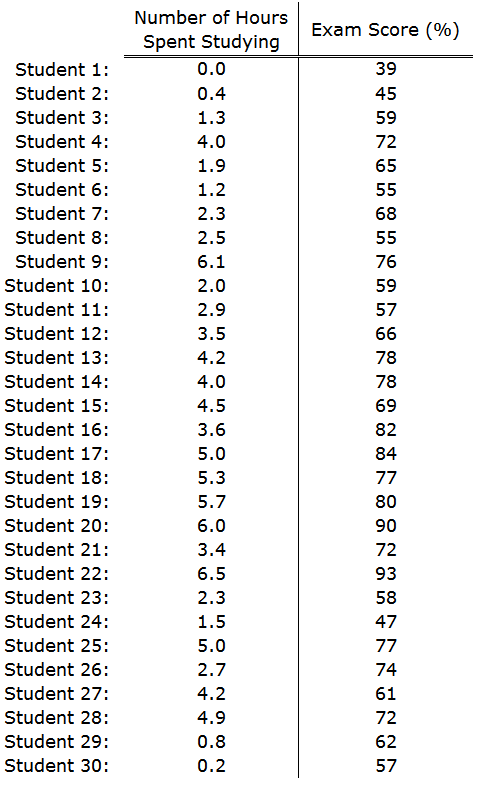To unlock this lesson you must be a Study.com Member.

### Register to view this lesson

Are you a student or a teacher?

### Unlock Your Education

#### See for yourself why 30 million people use Study.com

##### Become a Study.com member and start learning now.
Back
What teachers are saying about Study.com

### Earning College Credit

Did you know… We have over 160 college courses that prepare you to earn credit by exam that is accepted by over 1,500 colleges and universities. You can test out of the first two years of college and save thousands off your degree. Anyone can earn credit-by-exam regardless of age or education level.